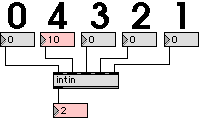# Digital Music Programming II: intin

This lab demonstrates how to get multiple integer inputs into a Max object.

Max enumerates the inputs to an object in an unusual way. The first inlet is handled differently than the other inlets, but you can consider it to be inlet 0. The other inlets are numbered from right to left starting with 1 on the far right.There are two steps to creating an inlet. First you must create the graphical inlets on the Max object. This is done in the create_object in the program code example below by calling the Max function intin. The program example needs to create 4 additional inputs, since the first input (inlet 0) is implicitly created.

```   intin(mo, 1);
intin(mo, 2);
intin(mo, 3);
intin(mo, 4);
```

Once you have defined inputs with the intin function, you can use the addinx and addint function to associate functions with inlets. Here is how this is done in the main function:

```   addint ((method)Input1);
```

The following program will generate an object with 5 inputs. Inside the code, the addinx functions will enumerate the inputs as 0, 4, 3, 2, 1 from left to right, but in the Max environment, the numbers 1, 2, 3, 4, 5 will be output when one of the respective inputs receives a number. For example, inlet 4 in the picture above received a value of 10 from a number box attached to it. This causes the number 2 to be output from the object because the second input to the object was triggered. (inlet 4 = input 2).

intin.c
 ``` #include "ext.h" typedef struct { t_object max_data; void* output; } MyObject; void* object_data = NULL; void main (void); void* create_object (void); void Input1 (MyObject* mo, long value); void Input2 (MyObject* mo, long value); void Input3 (MyObject* mo, long value); void Input4 (MyObject* mo, long value); void Input5 (MyObject* mo, long value); void main(void) { setup((t_messlist**)&object_data, (method)create_object, NULL, sizeof(MyObject), NULL, A_NOTHING); addint ((method)Input1); addinx ((method)Input2, 4); addinx ((method)Input3, 3); addinx ((method)Input4, 2); addinx ((method)Input5, 1); } void* create_object(void) { MyObject* mo = (MyObject*)newobject(object_data); mo->output = intout(mo); intin(mo, 1); intin(mo, 2); intin(mo, 3); intin(mo, 4); return mo; } void Input1(MyObject* mo, long value) { outlet_int(mo->output, 1); } void Input2(MyObject* mo, long value) { outlet_int(mo->output, 2); } void Input3(MyObject* mo, long value) { outlet_int(mo->output, 3); } void Input4(MyObject* mo, long value) { outlet_int(mo->output, 4); } void Input5(MyObject* mo, long value) { outlet_int(mo->output, 5); } ```

# Exercises

1. Compile the intin.c program to create a Max object. Run the object and verify that the output number relates to the input position in the patch.

2. Add another int inlet to the Max object. Keep the output numbers increasing from 1 on the left to 6 on the right.

3. Add a second output to the object which pass through the most recent input value.
```

```